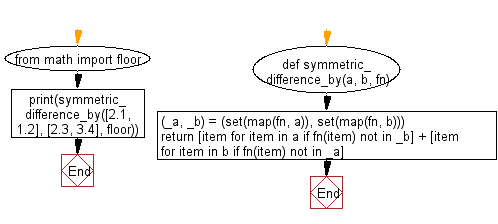﻿ Python: Symmetric difference between two lists, after applying the provided function to each list element of both - w3resource# Python: Symmetric difference between two lists, after applying the provided function to each list element of both

## Python List: Exercise - 227 with Solution

Write a Python program to get the symmetric difference between two lists, after applying the provided function to each list element of both.

• Create a set by applying fn to each element in every list.
• Use a list comprehension in combination with fn on each of them to only keep values not contained in the previously created set of the other.

Sample Solution:

Python Code:

``````def symmetric_difference_by(a, b, fn):
(_a, _b) = (set(map(fn, a)), set(map(fn, b)))
return [item for item in a if fn(item) not in _b] + [item
for item in b if fn(item) not in _a]
from math import floor
print(symmetric_difference_by([2.1, 1.2], [2.3, 3.4], floor))
```
```

Sample Output:

```[1.2, 3.4]
```

Flowchart:## Visualize Python code execution:

The following tool visualize what the computer is doing step-by-step as it executes the said program:

Python Code Editor:

Have another way to solve this solution? Contribute your code (and comments) through Disqus.

What is the difficulty level of this exercise?

Test your Python skills with w3resource's quiz

﻿

## Python: Tips of the Day

Floor Division:

When we speak of division we normally mean (/) float division operator, this will give a precise result in float format with decimals.

For a rounded integer result there is (//) floor division operator in Python. Floor division will only give integer results that are round numbers.

```print(1000 // 300)
print(1000 / 300)```

Output:

```3
3.3333333333333335```